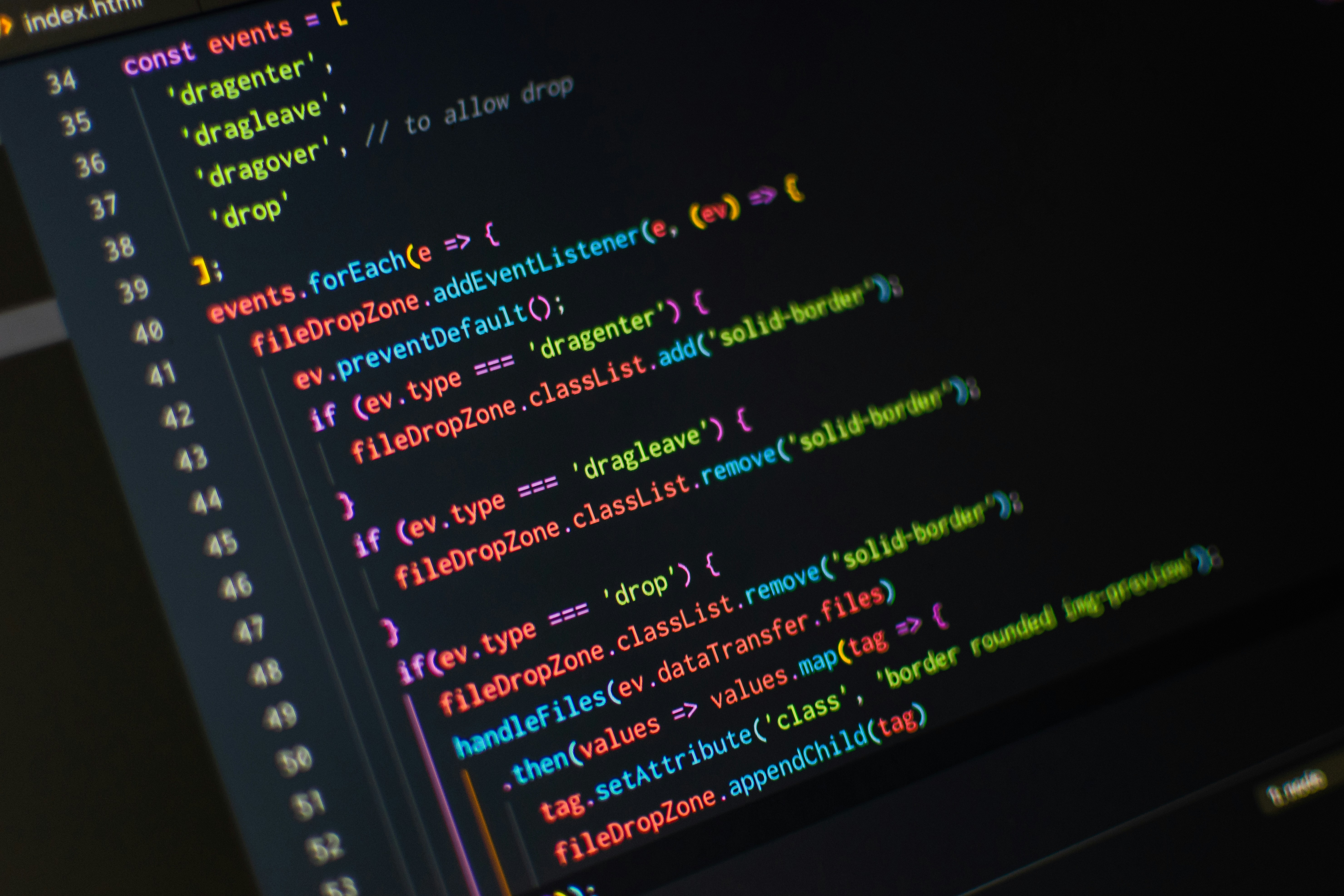# C++ 5. Memory ConceptsIn part 4, we created our first useful, awesome program. The Pythagorean Theorem Calculator. In this article I want to explain memory concepts very briefly.

When we write something like:

``int hello = 2;``

and run the code, the variable hello is assigned a memory location by the compiler, and in that location it will place the integer 2. At some point in our code we might change hello to 10:

``hello = 10;``

This will overwrite the previous value in that location. Notice how we only tell the computer once that we want the variable hello to have the data type integer. You don't have to type int everytime you use it, the computer remember that it's an integer. A variable have a name, type, size and a value.

There's not much more to say about it, but it's good to know how this works.

In the next article I will go through basic arithmetic. Read C++ 6. Basic Arithmetic here.